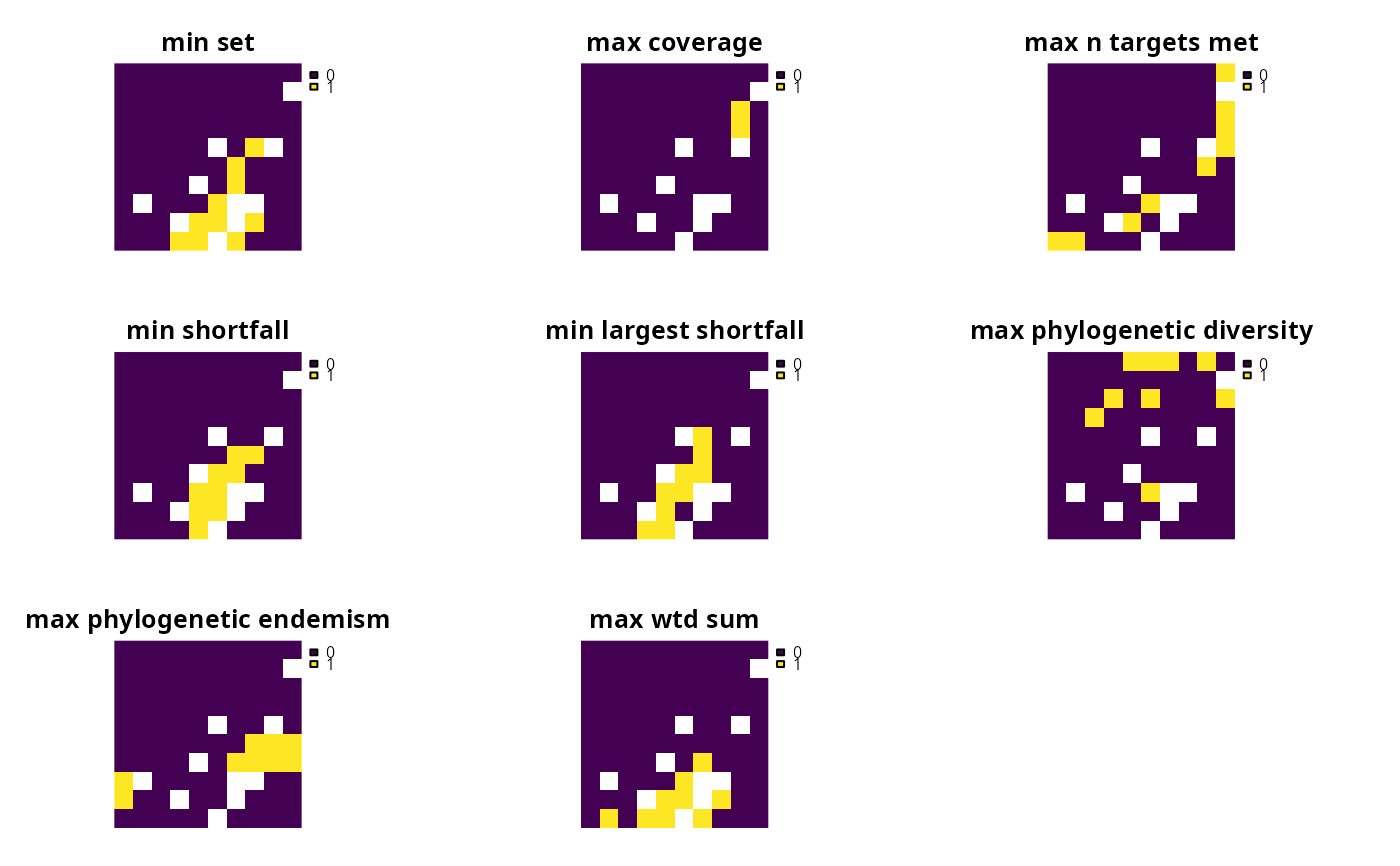An objective is used to specify the overall goal of a conservation planning problem. All conservation planning problems involve minimizing or maximizing some kind of objective. For instance, the planner may require a solution that conserves enough habitat for each species while minimizing the overall cost of the reserve network. Alternatively, the planner may require a solution that maximizes the number of conserved species while ensuring that the cost of the reserve network does not exceed the budget.

## Details

Please note that all conservation planning problems formulated using the prioritizr package require an objective function, and attempting to solve a problem without an objective will result in an error.

The following objectives can be added to a conservation planning problem():

add_min_set_objective()

Minimize the cost of the solution whilst ensuring that all targets are met. This objective is similar to that used in Marxan.

add_max_cover_objective()

Represent at least one instance of as many features as possible within a given budget.

add_max_features_objective()

Fulfill as many targets as possible while ensuring that the cost of the solution does not exceed a budget.

add_min_shortfall_objective()

Minimize the overall (weighted sum) shortfall for as many targets as possible while ensuring that the cost of the solution does not exceed a budget.

add_min_largest_shortfall_objective()

Minimize the largest (maximum) shortfall among all targets while ensuring that the cost of the solution does not exceed a budget.

add_max_phylo_div_objective()

Maximize the phylogenetic diversity of the features represented in the solution subject to a budget.

add_max_phylo_end_objective()

Maximize the phylogenetic endemism of the features represented in the solution subject to a budget.

add_max_utility_objective()

Secure as much of the features as possible without exceeding a budget.

Other overviews: constraints, decisions, importance, penalties, portfolios, solvers, summaries, targets

## Examples

# \dontrun{
sim_pu_raster <- get_sim_pu_raster()
sim_features <- get_sim_features()
sim_phylogeny <- get_sim_phylogeny()

# create base problem
p <-
problem(sim_pu_raster, sim_features) %>%

# create problem with added minimum set objective

# create problem with added maximum coverage objective
# note that this objective does not use targets

# create problem with added maximum feature representation objective

# create problem with added minimum shortfall objective

# create problem with added minimum largest shortfall objective

# create problem with added maximum phylogenetic diversity objective
p6 <- p %>% add_max_phylo_div_objective(1900, sim_phylogeny)

# create problem with added maximum phylogenetic diversity objective
p7 <- p %>% add_max_phylo_end_objective(1900, sim_phylogeny)

# create problem with added maximum utility objective
# note that this objective does not use targets

# solve problems
s <- c(
solve(p1), solve(p2), solve(p3), solve(p4), solve(p5), solve(p6),
solve(p7), solve(p8)
)
#> Warning: Targets specified for the problem will be ignored.
#> ℹ If the targets are important, use a different objective.
#> Warning: Targets specified for the problem will be ignored.
#> ℹ If the targets are important, use a different objective.
names(s) <- c(
"min set", "max coverage", "max features", "min shortfall",
"min largest shortfall", "max phylogenetic diversity",
"max phylogenetic endemism", "max utility"
)
# plot solutions
plot(s, axes = FALSE)# }